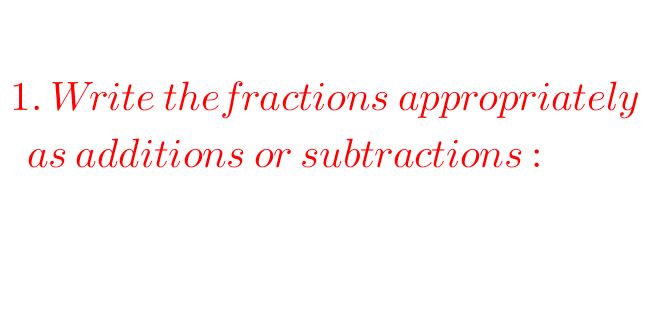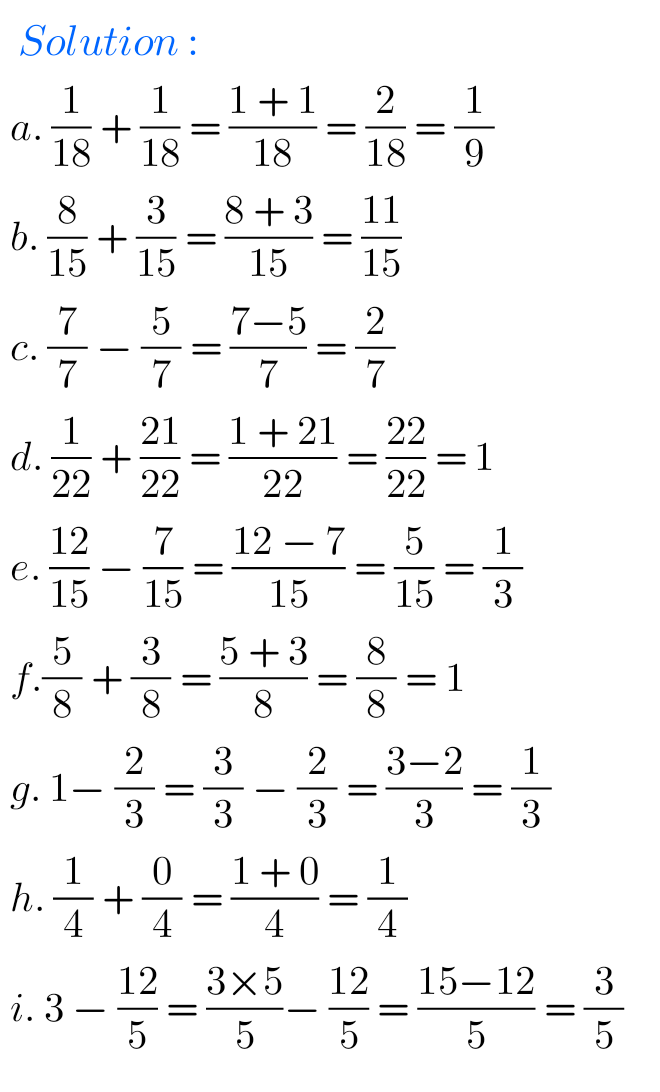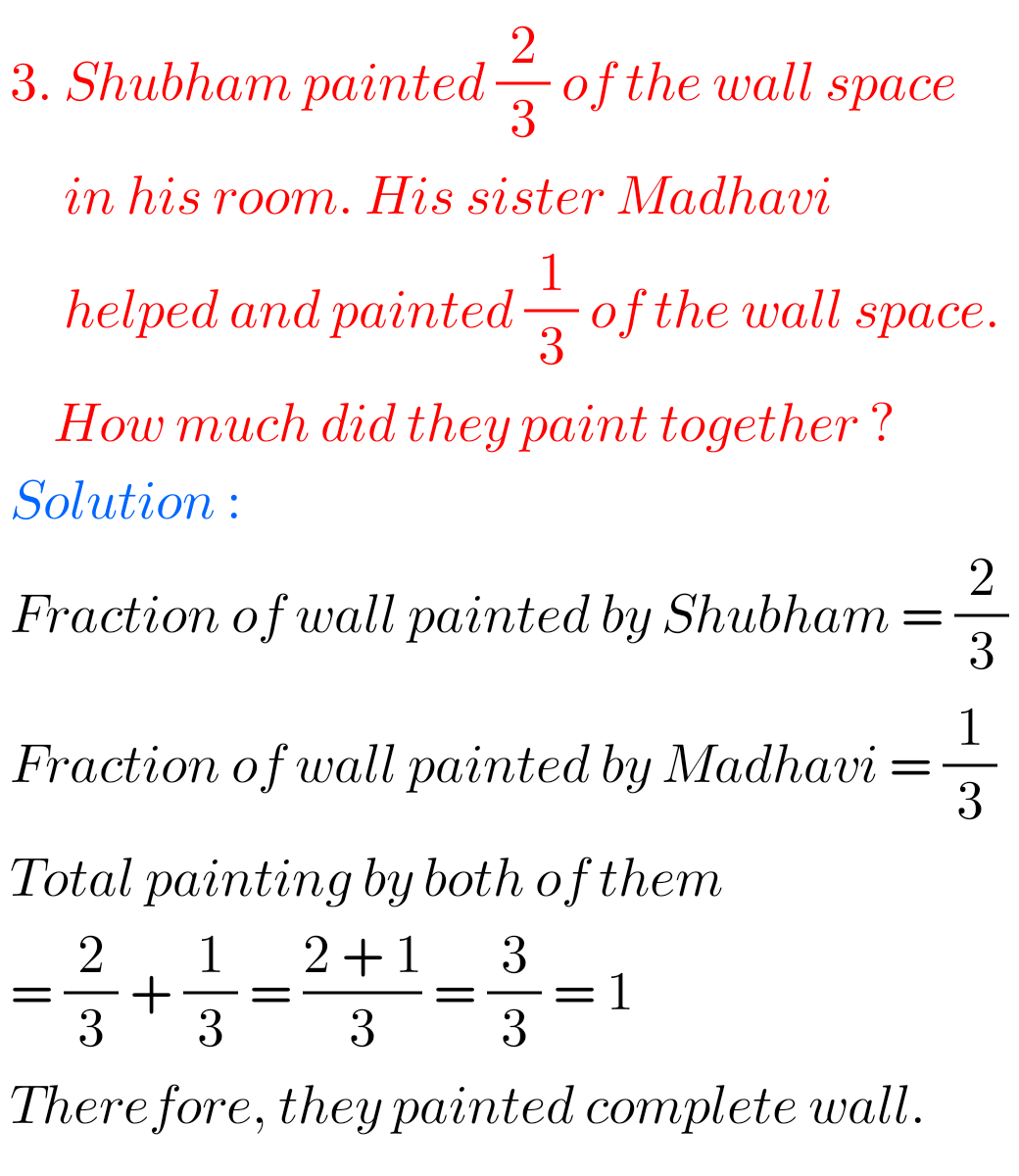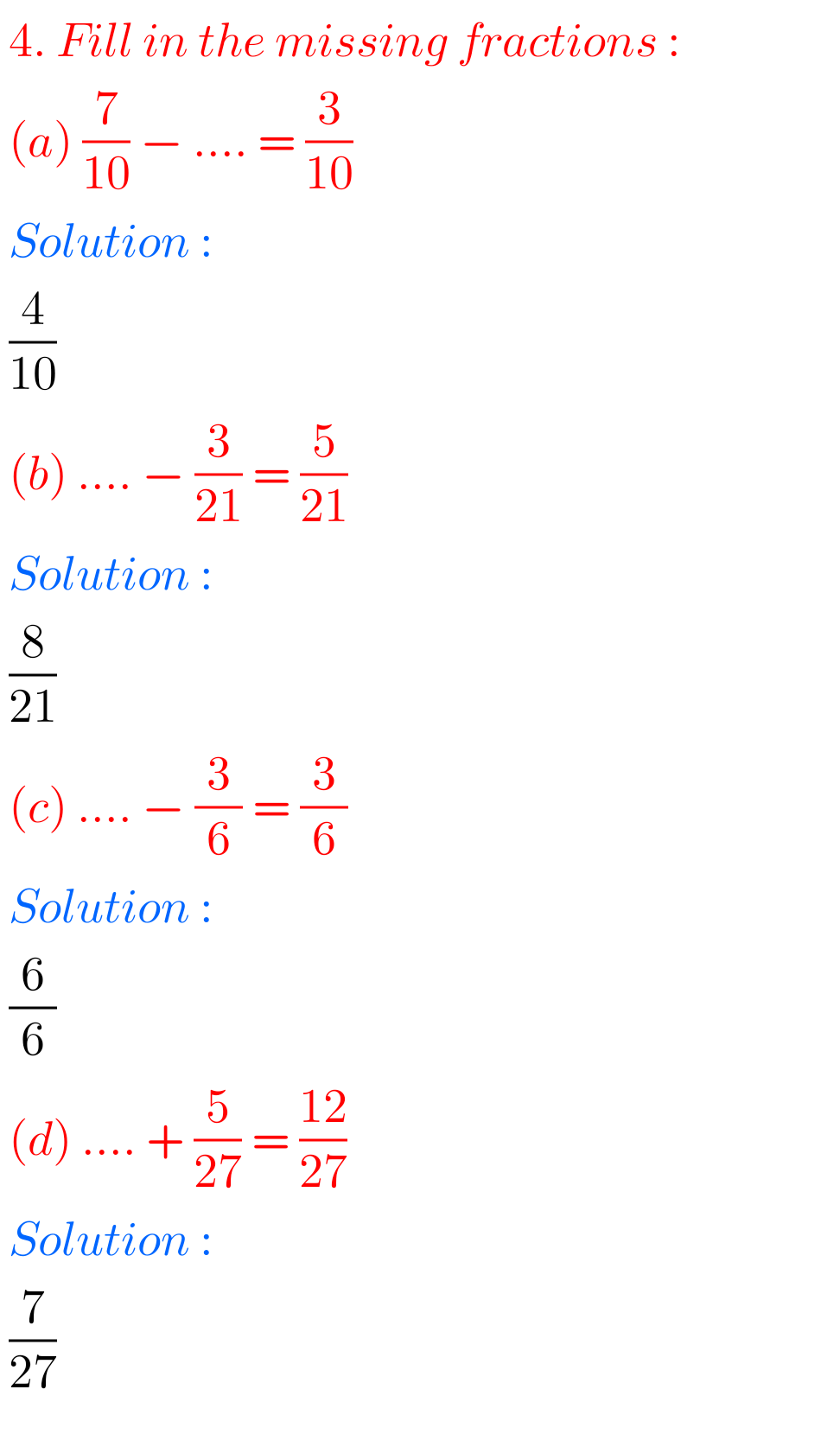# Maths Ncert class 6 Chapter 7 Fractions exercise 7.5 solutions

Solutions for Class 6 Ncert Maths chapter 7 Fractions exercise 7.5 are given.

First study the textbook lesson Fractions very well.

Observe the example problems and solutions given in the textbook. Try them well.

Observe the given below solutions and try them in your own method.

Ncert maths solutions for class 6
Fractions

Exercise 7.1

Exercise 7.2

Exercise 7.3

# 6th class Maths Ncert Chapter 7 Fractions exercise 7.5 solutions

Chapter -7     Fractions

Exercise 7.5F

F## Exercise 7.5 solutions Fractions Class 6 Ncert Maths## Solutions for Fractions Exercise 7.5 Class 6 Ncert MathsNote : Observe the solutions and tey them in your own method